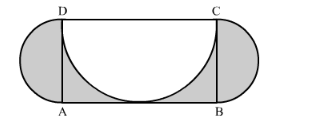# In the following figure, ABCD is a rectangle with AB = 14 cm and BC = 7 cm.

Question:

In the following figure, ABCD is a rectangle with AB = 14 cm and BC = 7 cm. Taking DCBC and AD as diameters, three semi-circles are drawn as shown in the figure. Find the area of the shaded region.Solution:

Area of the shaded region can be calculated as shown below,

Area of the shaded region = Area of rectangle − area of the semi-circle with diameter DC triangle + 2area of two semicircles with diameters AD and BC

$\therefore$ Area of the shaded region $=7 \times 14-\frac{\pi \times 7^{2}}{2}+2 \times \frac{\pi \times 3.5^{2}}{2}$

$\therefore$ Area of the shaded region $=98-\frac{\pi \times 49}{2}+\pi \times 12.25$

Substituting $\pi=\frac{22}{7}$ we get,

$\therefore$ Area of the shaded region $=98-\frac{\frac{22}{7} \times 49}{2}+\frac{22}{7} \times 12.25$

$\therefore$ Area of the shaded region $=98-\frac{22 \times 7}{2}+22 \times 1.75$

$\therefore$ Area of the shaded region $=98-77+22 \times 1.75$

$\therefore$ Area of the shaded region $=21+38.5$

$\therefore$ Area of the shaded region $=59.5$

Therefore, area of the shaded region is $59.5 \mathrm{~cm}^{2}$